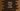# How to convert a set to tuple in Python## How to convert a set to tuple in Python:

We can easily convert a set to a tuple in Python. In python, we can easily change a set to tuple. In this post, I will show you how to convert a set to tuple with examples.

### What are set and tuple:

set and tuple, both are inbuilt data types in python. A set is used to hold unique items and it is mutable, i.e. we can change the items of a set. A tuple is immutable and it is ordered.

We can create a tuple by adding comma separated items in a () and a set by adding comma separated items inside a {}.

For example:

``````t = (1, 2, 3, 4, 3, 2, 1)
s = {1, 2, 3, 4, 3, 2, 1}

print(str(t))
print(str(s))``````

Here, t is a tuple and s is a set. If you run this, it will print the below output:

``````(1, 2, 3, 4, 3, 2, 1)
{1, 2, 3, 4}``````

As you can see, all duplicate items are removed from the set.

## How to convert a set to tuple:

We can convert a set to tuple easily. We need to pass the set to tuple() function and it is done. It will convert that set to a tuple. Let’s take a look at the below program:

``````s = {1, 2, 3, 4, 3, 2, 1}
t = tuple(s)

print(str(t))
print(str(s))``````

If you run this, it will print the below output:

``````(1, 2, 3, 4)
{1, 2, 3, 4}``````

As you can see here, the set is converted to a tuple.

## Example 2:

Let’s take another example:

``````s = {'hello', 'world', 1, 2, 3}
t = tuple(s)

print(str(t))
print(str(s))``````

It will print:

``````(1, 2, 3, 'hello', 'world')
{1, 2, 3, 'hello', 'world'}``````